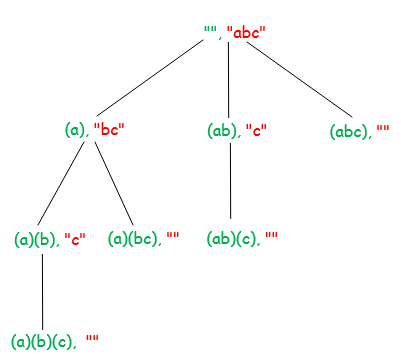Open In App

# Print all ways to break a string in bracket form

Given a string, find all ways to break the given string in bracket form. Enclose each substring within a parenthesis.

Examples:

```Input : abc
Output: (a)(b)(c)
(a)(bc)
(ab)(c)
(abc)

Input : abcd
Output : (a)(b)(c)(d)
(a)(b)(cd)
(a)(bc)(d)
(a)(bcd)
(ab)(c)(d)
(ab)(cd)
(abc)(d)
(abcd)```

We strongly recommend you to minimize your browser and try this yourself first.

The idea is to use recursion. We maintain two parameters – index of the next character to be processed and the output string so far. We start from index of next character to be processed, append substring formed by unprocessed string to the output string and recurse on remaining string until we process the whole string. We use std::substr to form the output string. substr(pos, n) returns a substring of length n that starts at position pos of current string.

Below diagram shows recursion tree for input string “abc”. Each node on the diagram shows processed string (marked by green) and unprocessed string (marked by red).Below is the implementation of the above idea

## C++

 `// C++ Program to find all combinations of Non-``// overlapping substrings formed from given``// string``#include ``using` `namespace` `std;` `// find all combinations of non-overlapping``// substrings formed by input string str``// index – index of the next character to``//          be processed``// out - output string so far``void` `findCombinations(string str, ``int` `index, string out)``{``    ``if` `(index == str.length())``        ``cout << out << endl;` `    ``for` `(``int` `i = index; i < str.length(); i++)``    ``{``        ``// append substring formed by str[index,``        ``// i] to output string``        ``findCombinations(``            ``str,``            ``i + 1,``            ``out + ``"("` `+ str.substr(index, i + 1 - index)``                ``+ ``")"``);``    ``}``}` `// Driver Code``int` `main()``{``    ``// input string``    ``string str = ``"abcd"``;` `    ``findCombinations(str, 0, ``""``);` `    ``return` `0;``}`

## Java

 `// Java program to find all combinations of Non-``// overlapping substrings formed from given``// string` `class` `GFG``{``    ``// find all combinations of non-overlapping``    ``// substrings formed by input string str``    ``static` `void` `findCombinations(String str, ``int` `index,``                                 ``String out)``    ``{``        ``if` `(index == str.length())``            ``System.out.println(out);`` ` `        ``for` `(``int` `i = index; i < str.length(); i++)`` ` `            ``// append substring formed by str[index,``            ``// i] to output string``            ``findCombinations(str, i + ``1``, out +``                ``"("` `+ str.substring(index, i+``1``) + ``")"` `);``    ``}``    ` `    ``// Driver Code``    ``public` `static` `void` `main (String[] args)``    ``{``        ``// input string``        ``String str = ``"abcd"``;``        ``findCombinations(str, ``0``, ``""``);``    ``}``}` `// Contributed by Pramod Kumar`

## Python3

 `# Python3 Program to find all combinations of Non-``# overlapping substrings formed from given``# string` `# find all combinations of non-overlapping``# substrings formed by input string str``# index – index of the next character to``#          be processed``# out - output string so far``def` `findCombinations(string, index, out):``    ``if` `index ``=``=` `len``(string):``        ``print``(out)` `    ``for` `i ``in` `range``(index, ``len``(string), ``1``):` `        ``# append substring formed by str[index,``        ``# i] to output string``        ``findCombinations(string, i ``+` `1``, out ``+` `"("` `+``                         ``string[index:i ``+` `1``] ``+` `")"``)` `# Driver Code``if` `__name__ ``=``=` `"__main__"``:` `    ``# input string``    ``string ``=` `"abcd"``    ``findCombinations(string, ``0``, "")` `# This code is contributed by``# sanjeev2552`

## C#

 `// C# program to find all combinations``// of Non-overlapping substrings formed``// from given string``using` `System;` `class` `GFG {``    ``// find all combinations of non-overlapping``    ``// substrings formed by input string str``    ``public` `static` `void``    ``findCombinations(``string` `str, ``int` `index, ``string` `@``out``)``    ``{``        ``if` `(index == str.Length) {``            ``Console.WriteLine(@``out``);``        ``}` `        ``for` `(``int` `i = index; i < str.Length; i++) {` `            ``// append substring formed by``            ``// str[index, i] to output string``            ``findCombinations(``                ``str, i + 1,``                ``@``out` `+ ``"("``                    ``+ str.Substring(index, (i + 1) - index)``                    ``+ ``")"``);``        ``}``    ``}` `    ``// Driver Code``    ``public` `static` `void` `Main(``string``[] args)``    ``{``        ``// input string``        ``string` `str = ``"abcd"``;``        ``findCombinations(str, 0, ``""``);``    ``}``}` `// This code is contributed by Shrikant13`

## Javascript

 `// Javascript program for the above approach` `// find all combinations of non-overlapping``// substrings formed by input string str``// index – index of the next character to``//          be processed``// out - output string so far``function` `findCombinations(string, index, out) {``  ``if` `(index == string.length) {``    ``console.log(out);``  ``}` `  ``for` `(let i = index; i < string.length; i++) {``    ``// append substring formed by str[index,``    ``// i] to output string``    ``findCombinations(string, i + 1, out + ``"("` `+ string.substring(index, i + 1) + ``")"``);``  ``}``}` `// Driver Code``const string = ``"abcd"``;``findCombinations(string, 0, ``""``);`  `// contributed by adityasharmadev01`

Output

```(a)(b)(c)(d)
(a)(b)(cd)
(a)(bc)(d)
(a)(bcd)
(ab)(c)(d)
(ab)(cd)
(abc)(d)
(abcd)```

Time Complexity: O(N2)
Auxiliary Space: O(N2)